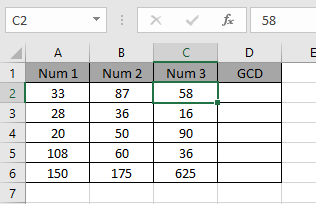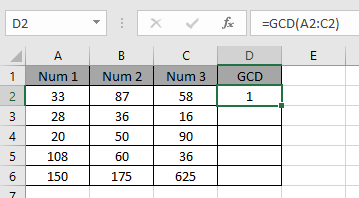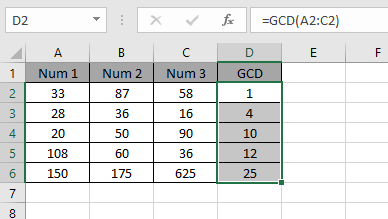# How to use the GCD Function in Excel

GCD is Greatest common divisor. GCD of two or more integers is the product of all the common divisors of the input values. It is commonly known as HCF full form is Highest Common Factor

Let’s take an example to see how GCD function works.
Let’s first take two number 45 & 54. The factors for the numbers are:

45 : 1, 3, 3, 5, 45
54 : 1, 3, 3, 3, 2, 54

The common divisors from the above 2 factors are:

3 x 3 =9

Multiply them to get the GCD of 45 & 54. Greatest Common Divisor is 9 for the two numbers.

The GCD function returns a number representing the GCD of the input numbers. It takes numbers as an argument and returns the return GCD of the value using the formula.
Syntax:

=GCD (number1, [number2])

Arguments to the function can be given an array on directly supplied integers.

Let’s understand the GCD function via using it on some numbers to get results.To get the GCD or HCF for the number shown in the above snapshot.

Use the formula:

=GCD(A2:C2)

Numbers provided in as arrayIf there is no common divisor between the factors of the numbers, the formula returns 1 because 1 is a factor of every integer.As you can see the Highest common factor or GCD of the numbers is calculated using the formula.

Hope you understood how to use the GCD function in Excel. Explore more articles on Excel mathematical functions here. Please feel free to state your query or feedback for the above article.

Related Articles:

How to use the LCM Function in Excel

Popular Articles:

50 Excel Shortcuts to Increase Your Productivity

How to use the VLOOKUP Function in Excel

How to use the COUNTIF in Excel 2016

How to use the SUMIF Function in Excel

Terms and Conditions of use

The applications/code on this site are distributed as is and without warranties or liability. In no event shall the owner of the copyrights, or the authors of the applications/code be liable for any loss of profit, any problems or any damage resulting from the use or evaluation of the applications/code.